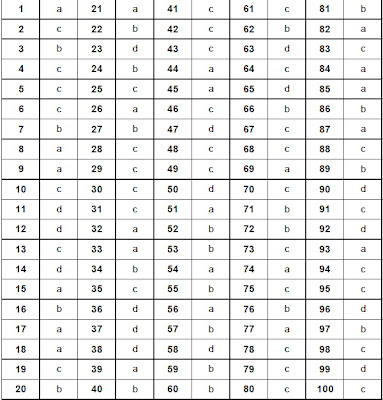## Saturday, January 28, 2012

### SCRA 2012 Answer key And Cutoff MarksThe answer keys of Special Class Railway Apprentices(SCRA) exam held on 29 January 2012 by Union Public Service Commission (UPSC) for the post of Mechanical Engineers in Indian Railway can be seen now

The solutions of questions paper Code A can be seen below.Paper was of moderate difficulty and students had a huge opportunity of raising there score in the General Ability Test.

It is expected that a student scoring 50-65% of the maximum marks can clear the cutoff for this year.Though these numbers are just predictions on the basis of previous results.

The solution key for Test Booklet Series A (Set code 'A') are as follows:

### General Ability Test (Paper 1)

1. A 2. C 3.A 4.B 5.C

6. A 7. C 8. B 9. D 10. B

11. A 12. C 13. D 14. D 15. D

16. A 17.B 18.D 19.B 20.B

21. D 22. A 23. C 24.A 25.D

26. B 27. A 28. C 29. A 30.C

31. A 32. B 33. B 34. C 35. C

36. A 37. B 38. B 39. C 40. A

41. B 42. A 43. A 44. C 45. D

46. B 47. B 48. D 49. C 50. B

51. A 52. B 53. A 54. D 55. B

56. B 57. C 58. A 59. A 60. A

61. B 62. A 63. B 64. C 65. A

66. A 67. D 68. A 69. D 70. A

71. B 72. C 73. A 74. C 75. D

76. A 77. B 78. B 79. B 80. C

81. A 82. D 83. B 84. B 85. A

86. D 87. D 88. C 89. B 90.A

91. D 92. B 93. A 94. D 95. B

96. C 97. D 98. D 99. A 100. D

101. C 102. C 103. C 104. D 105. D

106. B 107. C 108. D 109. D 110. A

111. A 112. B 113. C 114. A 115. D

116. C 117 . C 118. D 119. C 120. C

### Physical Sciences(Paper 2)

1. d 21. c 41. a 61. d 81. a 101. d
2 .a 22. c 42. a 62. a 82. a 102. d
3. a 23. b 43. c 63. a 83. a 103. c
4. c 24. d 44. a 64. a 84. b 104. d
5.c 25. c 45. a 65. b 85. d 105. a
6. d 26. b 46. c 66. a 86. a 106. d
7. b 27. a 47. b 67. c 87. a 107. c
8. c 28. b 48. d 68. b 88. a 108. d
9. b 29. a 49. a 69. d 89. a 109. d
10. b 30. a 50. c 70. b 90. c 110. c
11. a 31. d 51. b 71. a 91. b 111. c
12. d 32. d 52. b 72. c 92. d 112. a
13. c 33. d 53. c 73. a 93. a 113. d
14. b 34. a 54. b 74. c 94. c 114. a
15. c 35. a 55. c 75. c 95. c 115. d
16. c 36. c 56. b 76. b 96. a 116. d
17. d 37. a 57. a 77. c 97. b 117. a
18. c 38. d 58. b 78. a 98. c 118. c
19. b 39. b 59. b 79. d 99. a 119. d
20. b 40. c 60. b 80. c 100. c 120. d

### Mathematics(Paper 3)

1. a 21. a 41. c 61. c 81. b
2. c 22. b 42. c 62. b 82. a
3. b 23. d 43. c 63. d 83. c
4. c 24. b 44. a 64. c 84. a
5. c 25. c 45. a 65. d 85. a
6. c 26. a 46. c 66. b 86. b
7. b 27. b 47. d 67. c 87. a
8. a 28. c 48. c 68. c 88. c
9. a 29. c 49. c 69. a 89. b
10. c 30. c 50. d 70. c 90. d
11. d 31. c 51. a 71. b 91. c
12. d 32. a 52. b 72. b 92. d
13. c 33. a 53. b 73. c 93. a
14. d 34. b 54. a 74. a 94. c
15. a 35. c 55. b 75. c 95. c
16. b 36. d 56. a 76. b 96. d
17. a 37. d 57. b 77. a 97. b
18. a 38. d 58. d 78. c 98. c
19. c 39. a 59. b 79. c 99. d
20. b 40. b 60. b 80. c 100. c

The Answer sheet can also be seen in the images below: (click on the image to zoom/download it)

Paper 2 Set APaper 3(Maths) Set ACompassionate heart said...

wen will rest ans will come??????

parth said...

plese give details of the sets then rint the answer ,they r wrong

Bimal Chandra Sinha said...

specify set.is it answers of set A/B/C/D?

ashok tak said...

when will result come

Unknown said...

what is expected marks for qualifying written part?????????????????